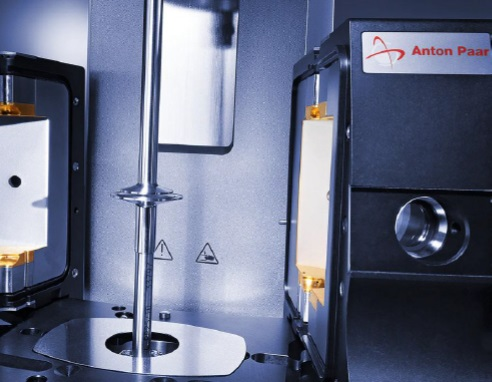# Calculation of the Weight Average Molar Mass Mw

The present application note explains how to calculate the molar mass Mw based on the Zero-Shear Viscosity value measured by a Frequency Sweep. The Zero-Shear Viscosity can be evaluated by applying the Carreau-Yasuda Regression to a Frequency Sweep. The tests were carried out on an MCR rheometer equipped with an Electrical Temperature Device.

## Introduction

The present application note explains how to calculate the molar mass Mw based on the Zero-Shear Viscosity value measured by a Frequency Sweep. The Zero-Shear Viscosity can be evaluated by applying the Carreau-Yasuda Regression to a Frequency Sweep.

## Experimental Setup

A technical, iso-tactic Polypropylene melt i-PP was measured using an MCR rheometer equipped with an Electrical Temperature Device consisting of basis plate and actively heated hood. The actively heated hood is based on a combination of radiation plus convection (N2,g) heating to prevent polymer degradation and to ensure precise and horizontally/vertically gradient free measurements at the set temperature of 200 °C.

The parallel plate measuring system PP25 was chosen and the measuring gap was set to 1 mm. To ensure a constant shear rate throughout the measuring gap we recommend using the TruGap measuring system CP25-3/TG (above 1000 Pas) or CP35-3/TG (below 1000 Pas) in combination with an MCR rheometer.

With respect to the linear visco-elastic range LVE-R a strain of 5 % was chosen. The angular frequency was varied logarithmically between 628 s-1 and 0.1 s-1 (100 Hz and 0.0159 Hz) with 5 measuring points per decade.Want to know more? Click here to read the full article.This information has been sourced, reviewed and adapted from materials provided by Anton Paar GmbH.

## Citations

• APA

Anton Paar GmbH. (2020, March 03). Calculation of the Weight Average Molar Mass Mw. AZoM. Retrieved on October 31, 2020 from https://www.azom.com/article.aspx?ArticleID=18956.

• MLA

Anton Paar GmbH. "Calculation of the Weight Average Molar Mass Mw". AZoM. 31 October 2020. <https://www.azom.com/article.aspx?ArticleID=18956>.

• Chicago

Anton Paar GmbH. "Calculation of the Weight Average Molar Mass Mw". AZoM. https://www.azom.com/article.aspx?ArticleID=18956. (accessed October 31, 2020).

• Harvard

Anton Paar GmbH. 2020. Calculation of the Weight Average Molar Mass Mw. AZoM, viewed 31 October 2020, https://www.azom.com/article.aspx?ArticleID=18956.